# Temperature Conversion Chart PrintableChartJan 07, 2022By PrintableeNo Comment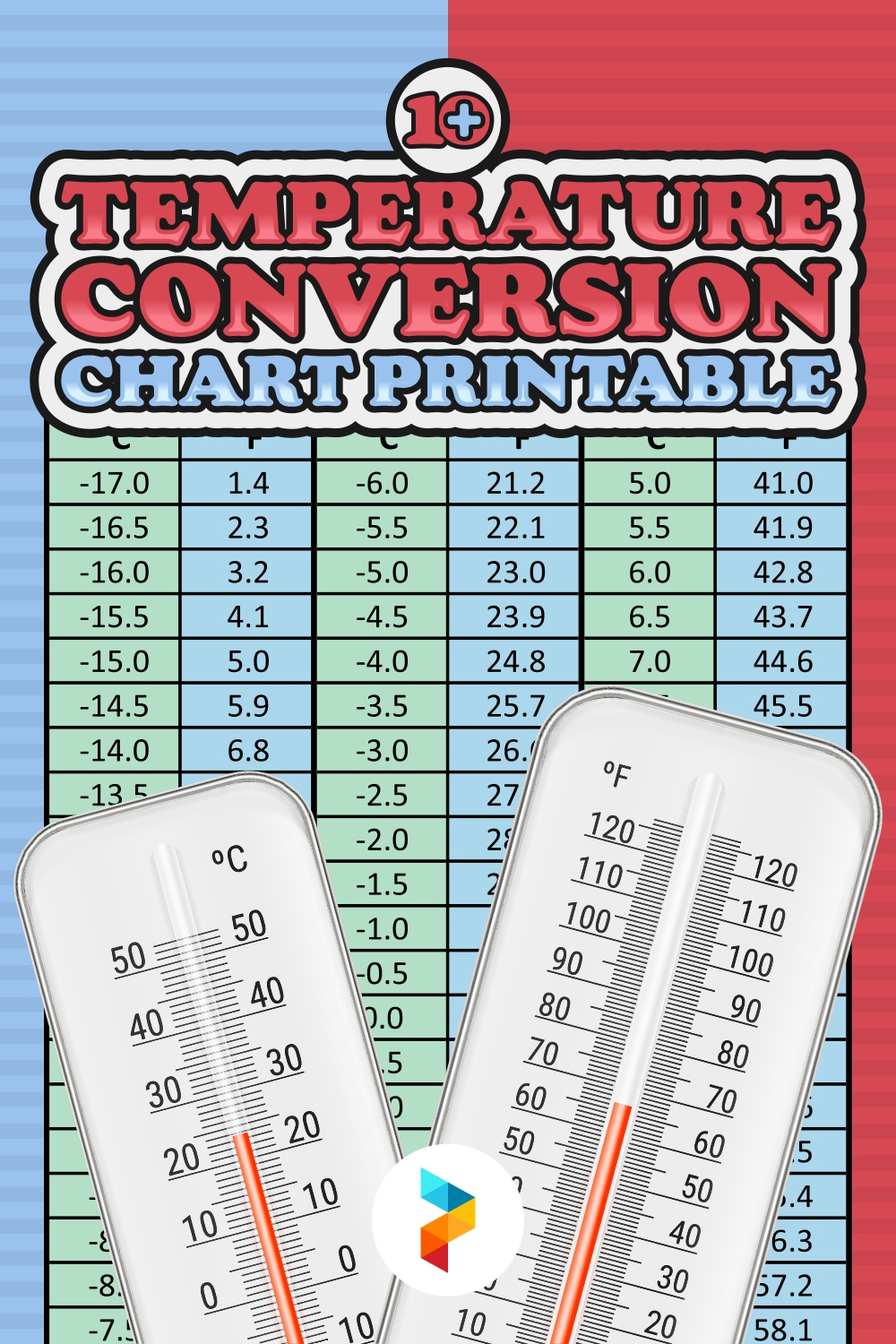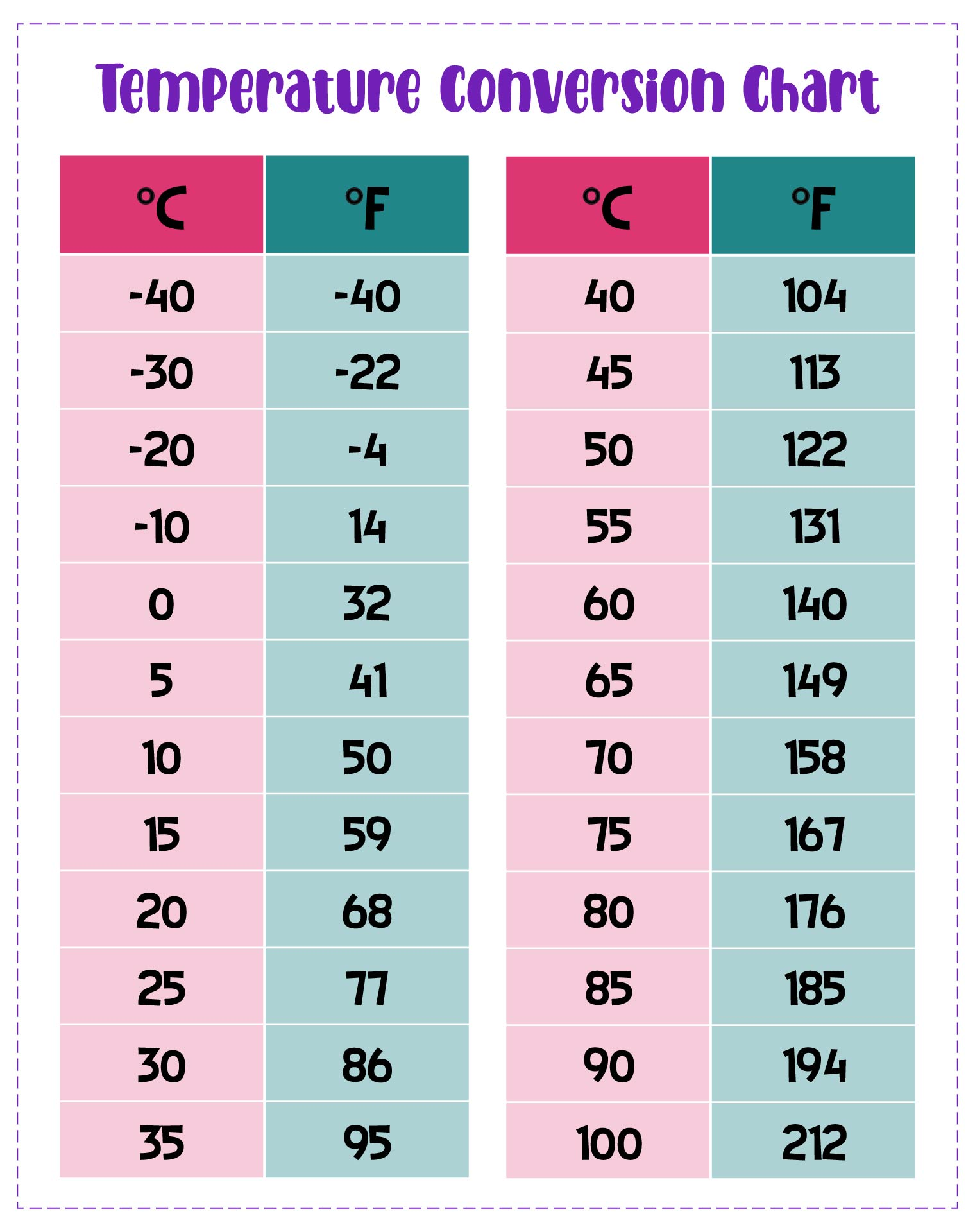### How do You Convert Fahrenheit to Celsius?

To convert temperatures in degrees Fahrenheit to Celsius, subtract 32 and multiply by .5556 (or 5/9). For example: (50°F – 32) x .5556 = 10°C.
If you don't want to count it yourself you can use the Fahrenheit to Celsius conversion chart. You can also use Fahrenheit to Celsius conversion calculator as a simple way to convert Fahrenheit to Celsius.

### How do You Convert Celsius to Fahrenheit?

To convert temperatures in degrees Celsius to Fahrenheit, you just do the opposite which is multiplied by 1.8 (or 9/5) and add 32. For example: (30°C x 1.8) + 32 = 86°F.
You can also use the Celsius to Fahrenheit chart or use a Celsius to Fahrenheit conversion calculator.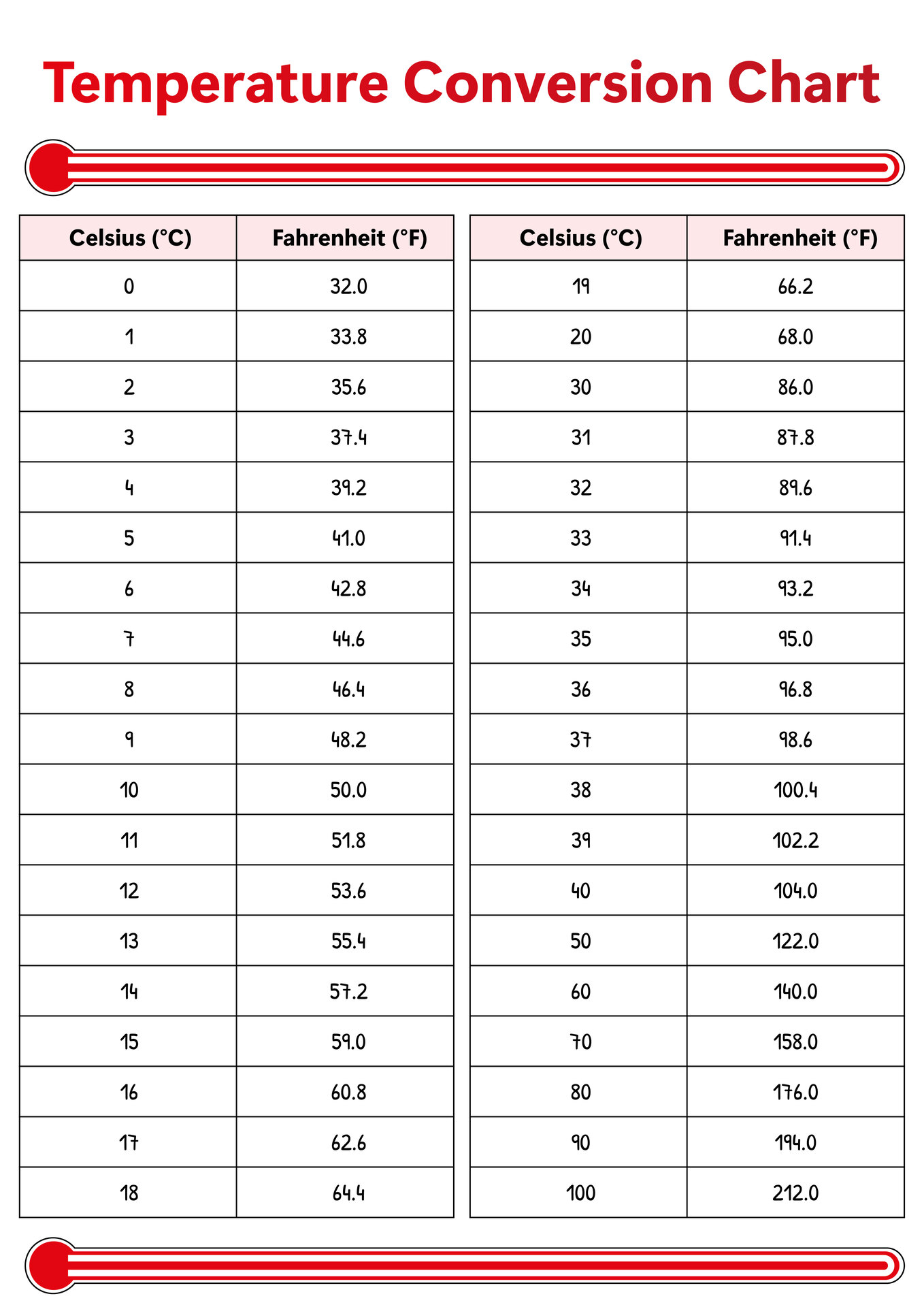We also have more printable chart you may like:
Printable Food Temperature Chart
Printable Vitamin And Mineral Chart
Printable Daily Mood Chart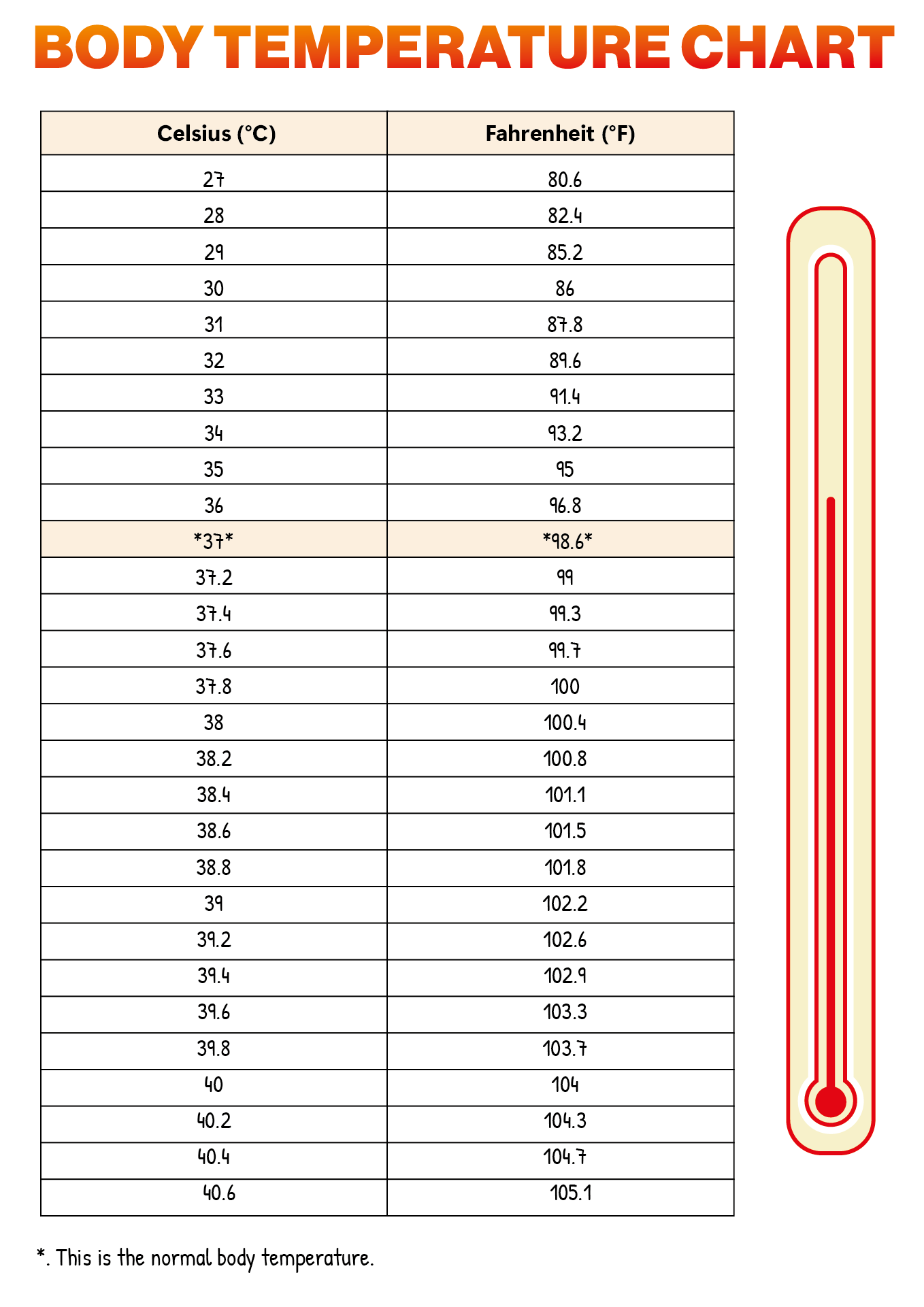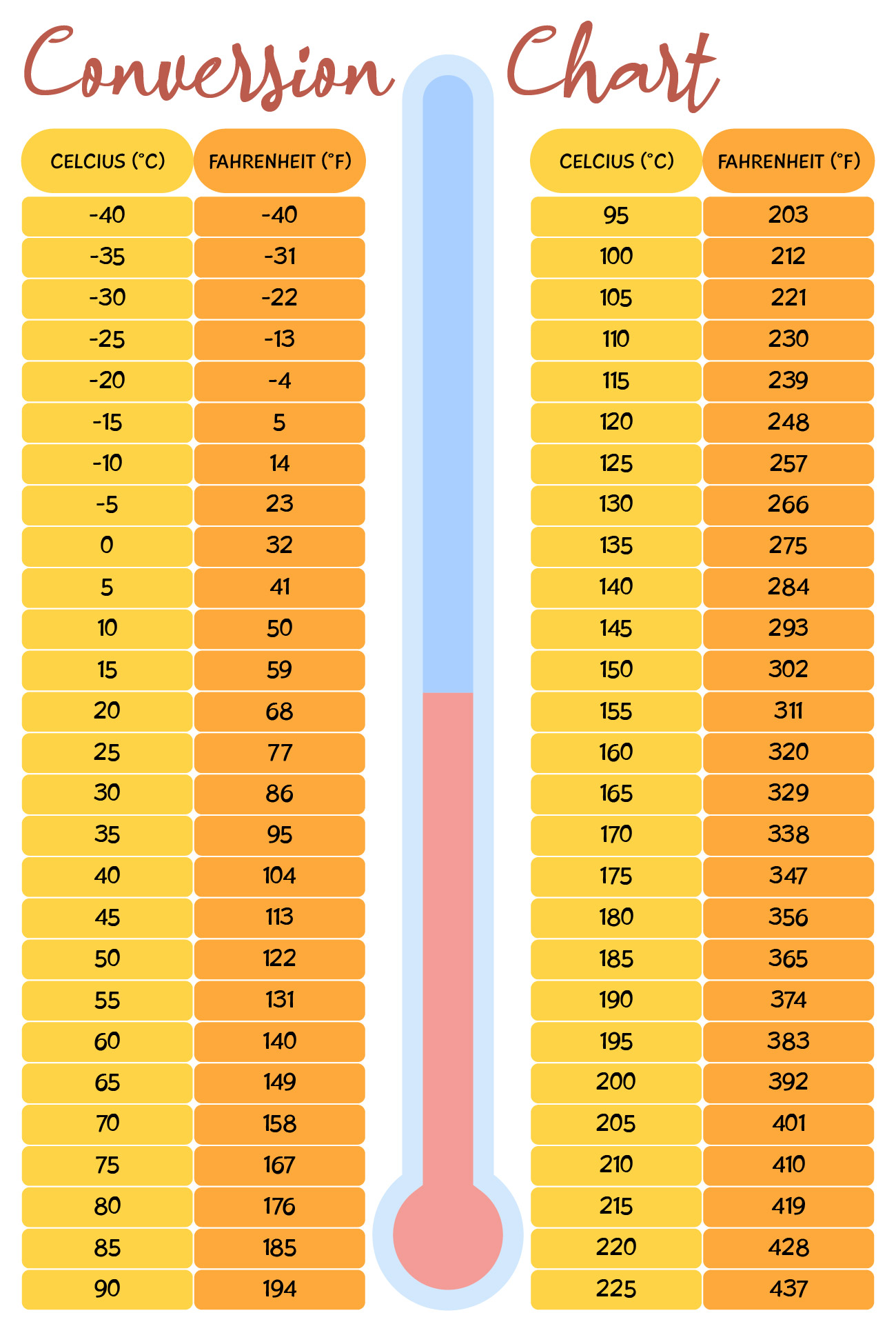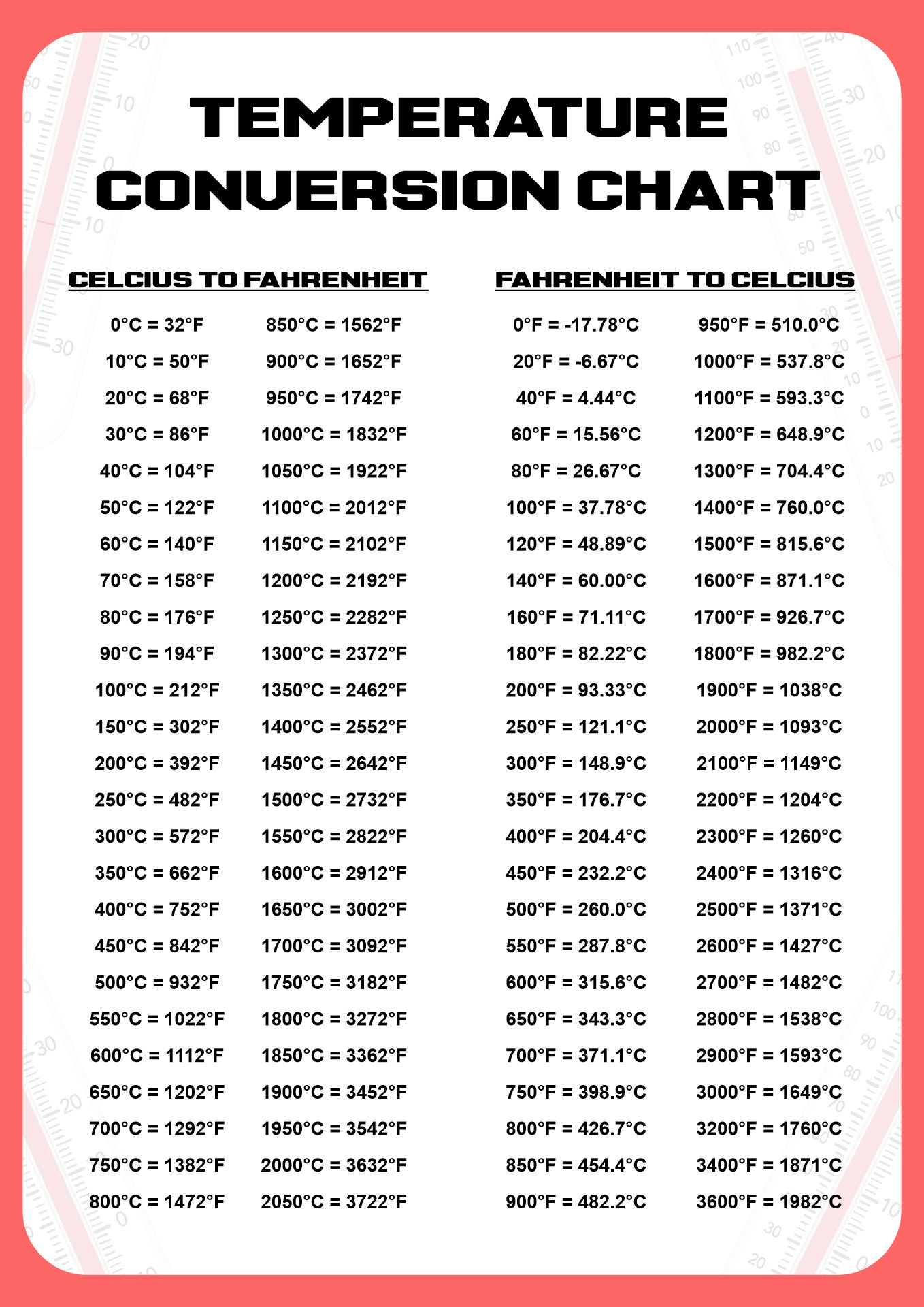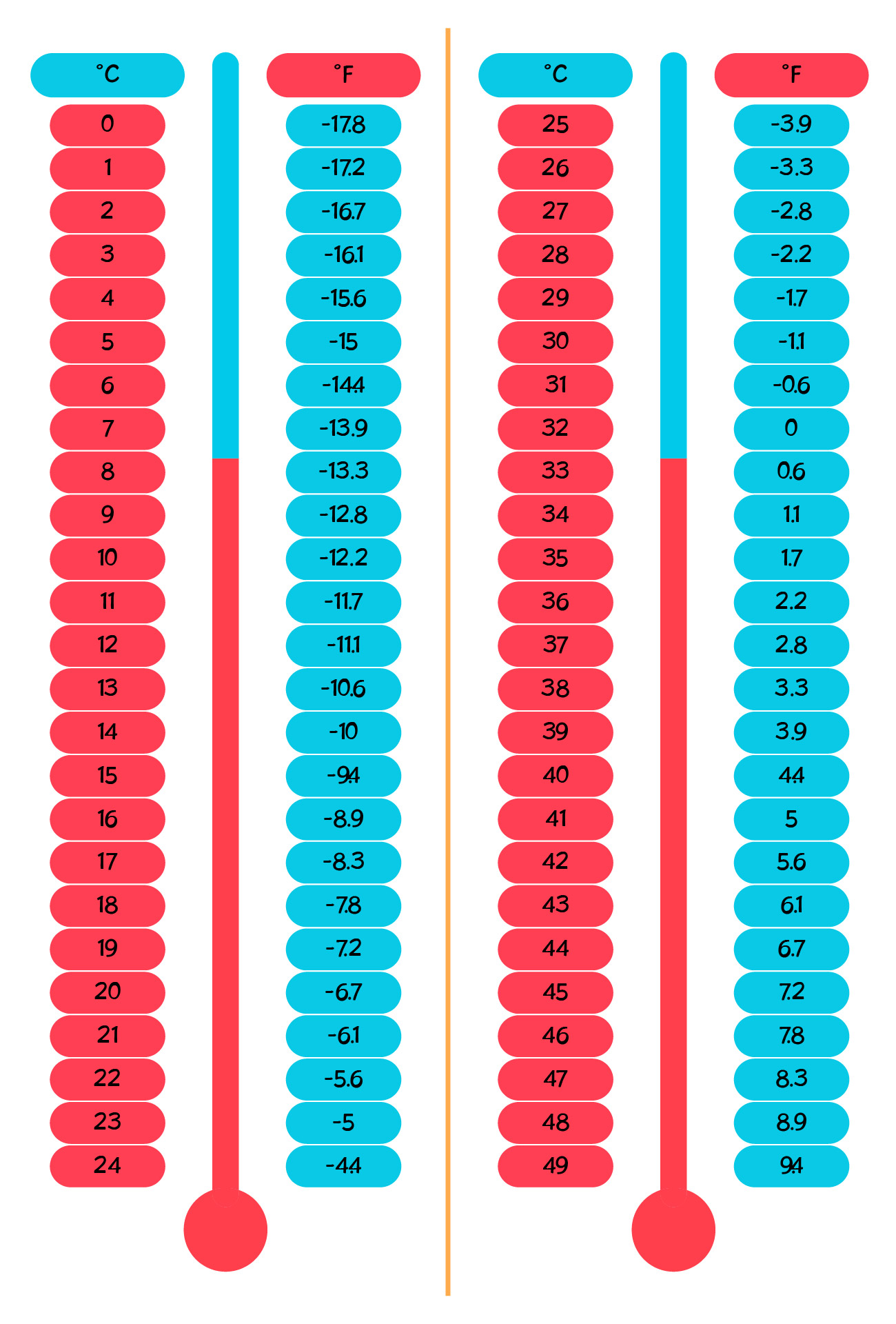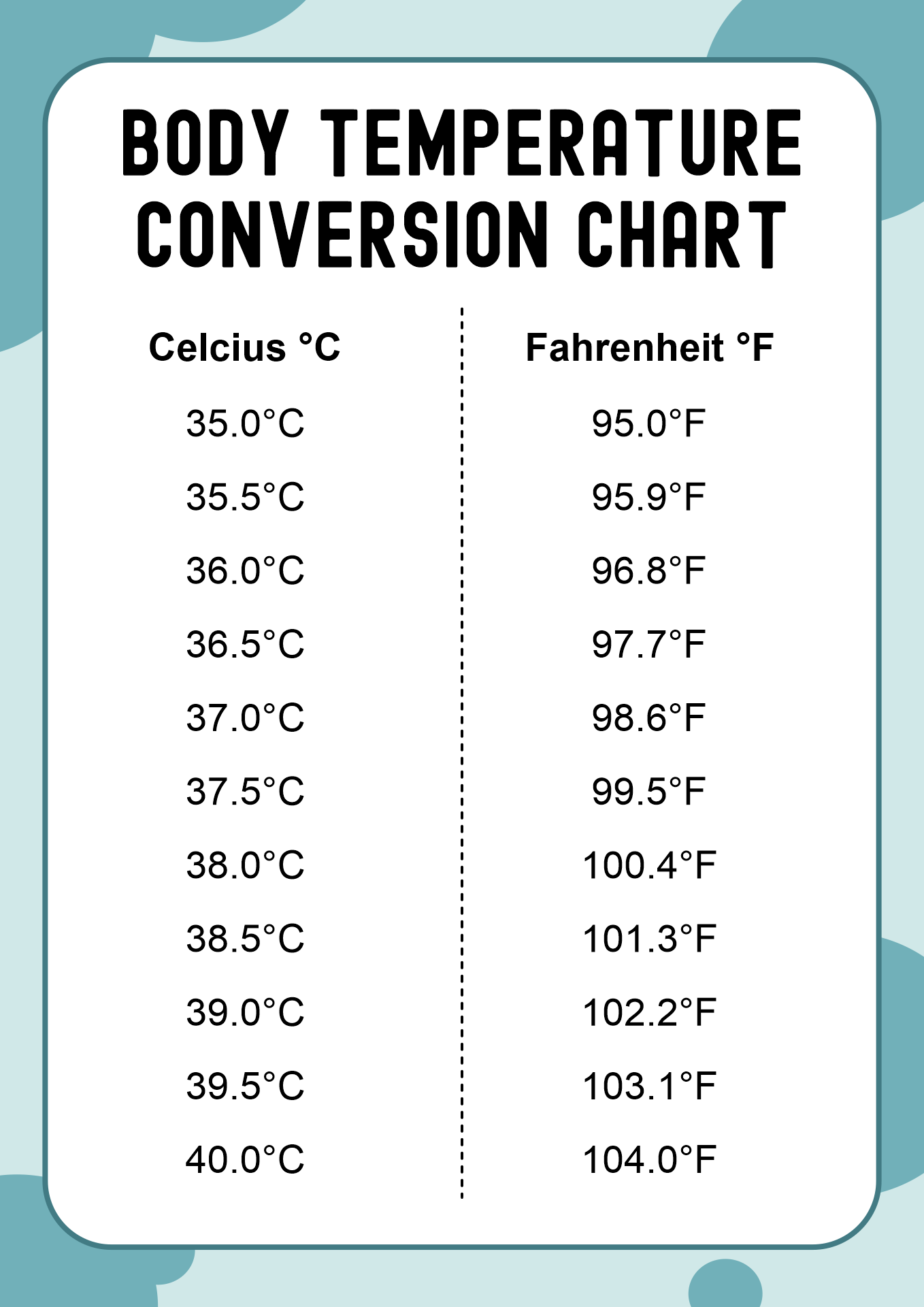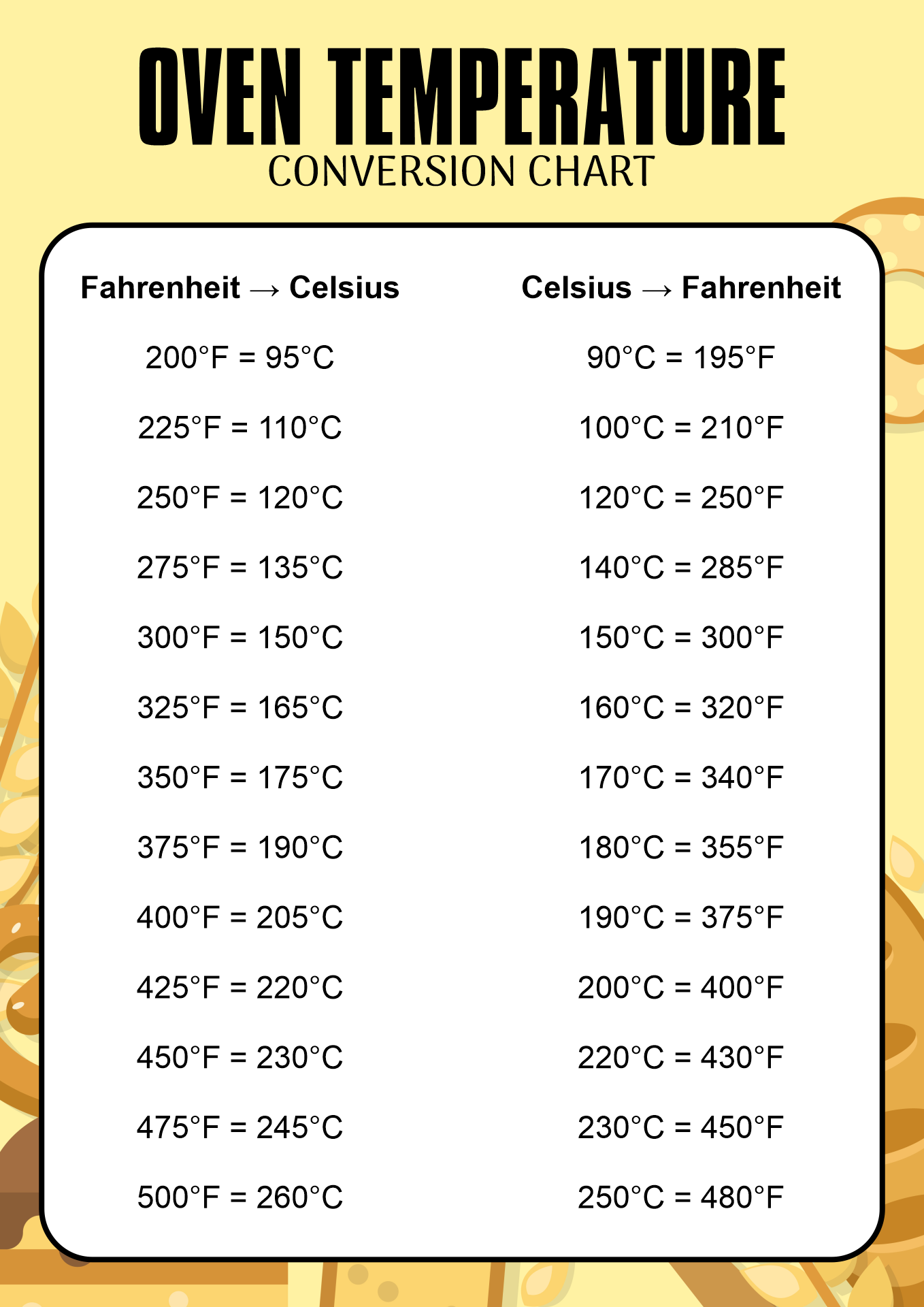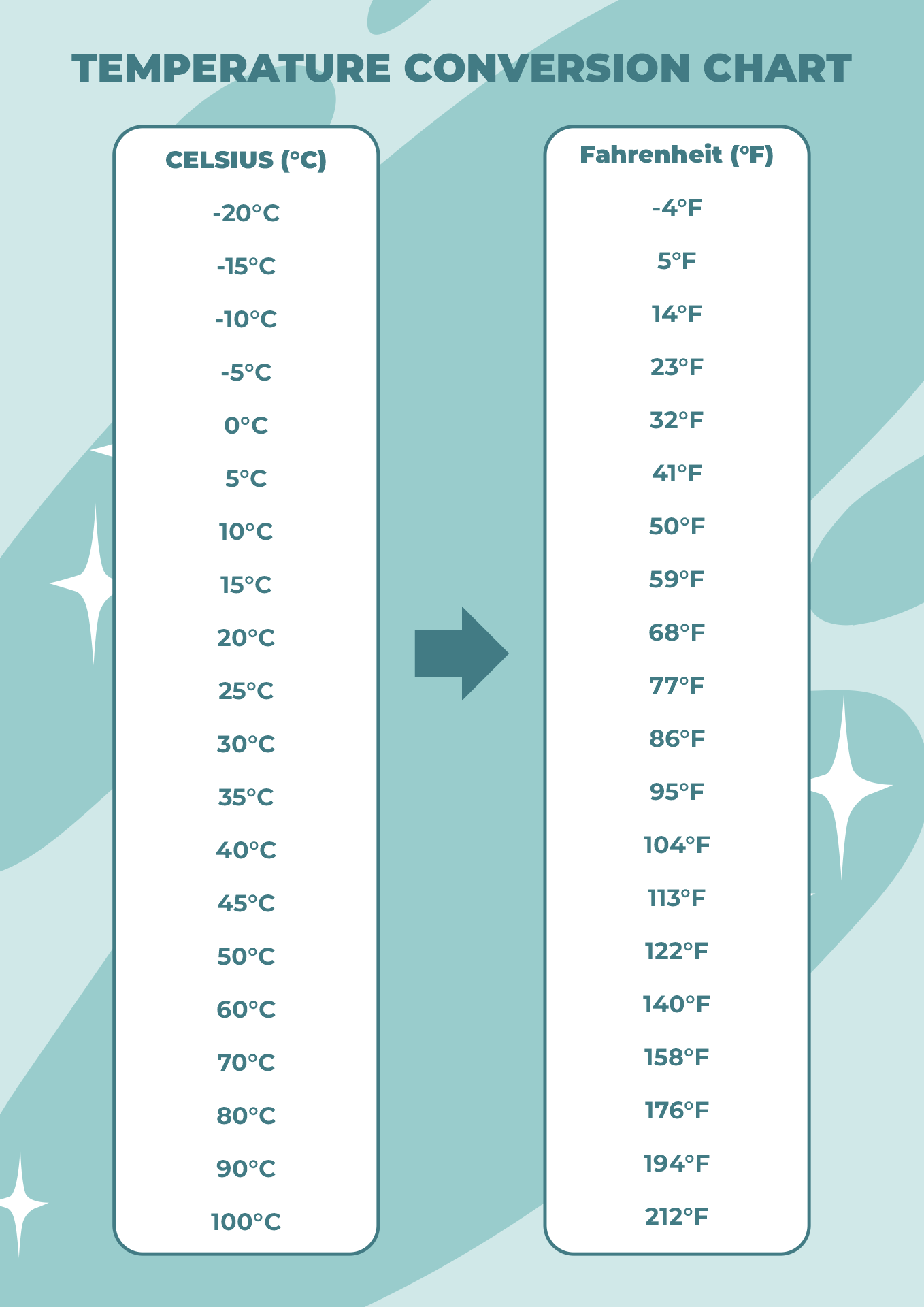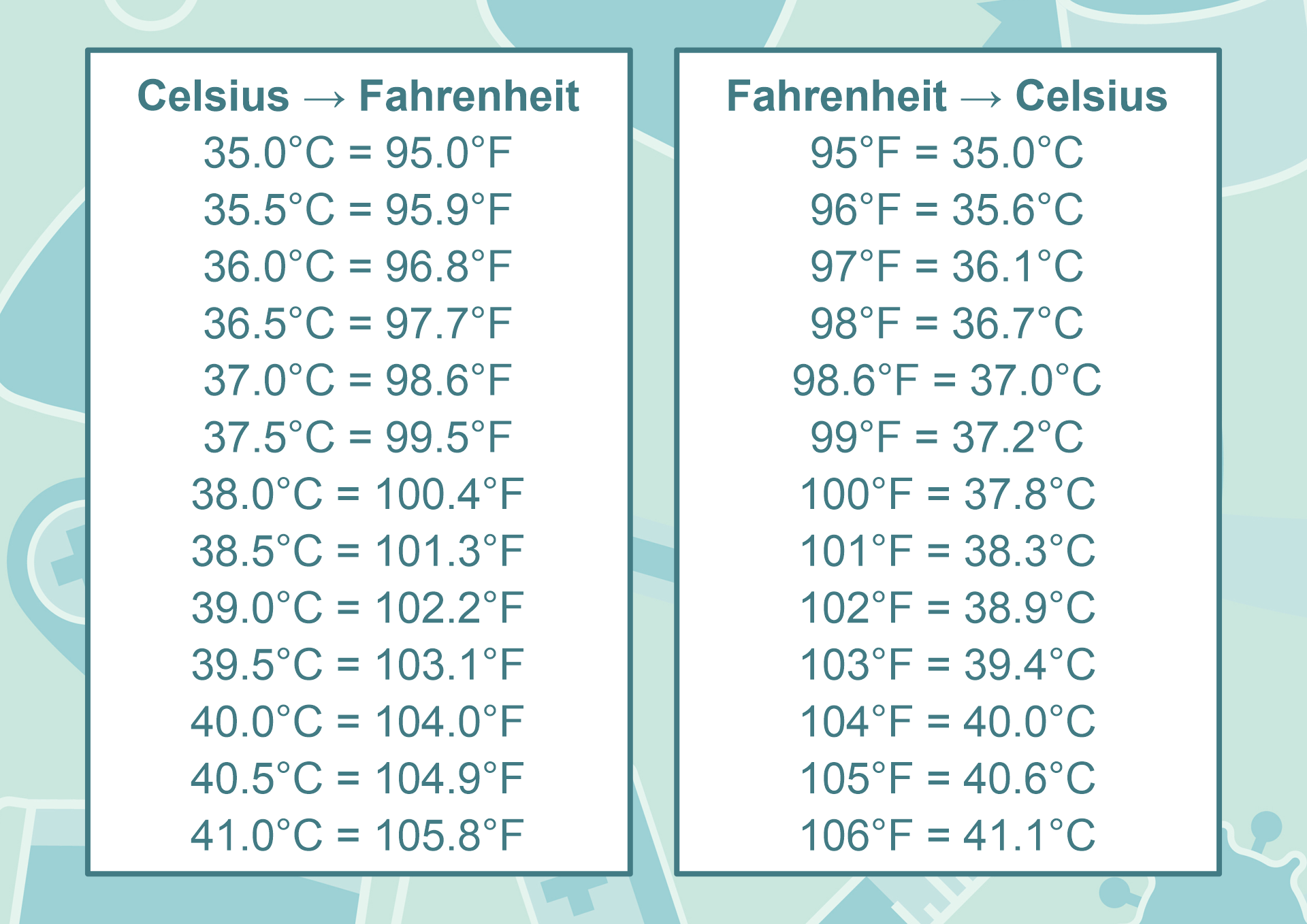### What are 5 Temperature Scales?

The 5 temperature scales are Celsius, Fahrenheit, Kelvin, Réaumur, and Rankine. Fahrenheit temperature scale is a scale based on 32 for the freezing point of water and 212 for the boiling point of water. Until the 1970s the Fahrenheit temperature scale was in general common use in English-speaking countries while the Celsius or centigrade scale was employed in most other countries worldwide. However, most English-speaking countries have officially adopted the Celsius scale.

Celsius temperature scale also called the centigrade temperature scale, is the scale based on 0 for the freezing point of water and 100 for the boiling point of water. Celsius is sometimes called the centigrade scale because of the 100-degree interval between the defined point.

Kelvin temperature scale is the base unit of thermodynamic temperature measurement in the International System (SI) of measurement. It is defined as 1/273.16 of the triple point (equilibrium among the solid, liquid, and gaseous phases) of pure water. The Kelvin scale has been adopted as the international standard for scientific temperature measurement. The Kelvin scale is related to the Celsius scale. The difference between the freezing point and boiling point of water is 100 degrees in each so that the Kelvin has the same magnitude as the degree Celsius.

The Réaumur scale is a temperature scale of which the freezing and boiling points of water are defined as 0 and 80 degrees respectively. The Réaumur scale was used widely in Europe, particularly in France, Germany, and Russia. By the 1790s, France had chosen the Celsius scale rather than the Reaumur measurement, but it was used commonly in some parts of Europe until at least the mid 19th century and in parts of Russia until the early 20th.

The Rankine scale is an absolute scale of thermodynamic temperature. It is used in engineering systems where heat computations are done using degrees Fahrenheit. Zero on both the Kelvin and Rankine scales is absolute zero, but a temperature difference of one Rankine degree is defined as equal to one Fahrenheit degree, rather than a Celsius degree used on the Kelvin scale.